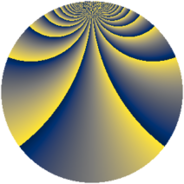# Properties

 Label 2240.2.fiLevel $2240$ Weight $2$ Character orbit 2240.fi Rep. character $\chi_{2240}(221,\cdot)$ Character field $\Q(\zeta_{48})$ Dimension $4096$ Sturm bound $768$

# Related objects

## Defining parameters

 Level: $$N$$ $$=$$ $$2240 = 2^{6} \cdot 5 \cdot 7$$ Weight: $$k$$ $$=$$ $$2$$ Character orbit: $$[\chi]$$ $$=$$ 2240.fi (of order $$48$$ and degree $$16$$) Character conductor: $$\operatorname{cond}(\chi)$$ $$=$$ $$448$$ Character field: $$\Q(\zeta_{48})$$ Sturm bound: $$768$$

## Dimensions

The following table gives the dimensions of various subspaces of $$M_{2}(2240, [\chi])$$.

Total New Old
Modular forms 6208 4096 2112
Cusp forms 6080 4096 1984
Eisenstein series 128 0 128

## Trace form

 $$4096q + O(q^{10})$$ $$4096q - 32q^{22} + 160q^{24} + 80q^{28} + 160q^{38} + 80q^{42} + 16q^{44} + 96q^{52} - 64q^{59} - 192q^{64} + 96q^{66} + 160q^{67} + 128q^{71} - 16q^{74} - 192q^{78} + 112q^{84} + 288q^{90} - 272q^{96} + 272q^{98} + O(q^{100})$$

## Decomposition of $$S_{2}^{\mathrm{new}}(2240, [\chi])$$ into newform subspaces

The newforms in this space have not yet been added to the LMFDB.

## Decomposition of $$S_{2}^{\mathrm{old}}(2240, [\chi])$$ into lower level spaces

$$S_{2}^{\mathrm{old}}(2240, [\chi]) \cong$$ $$S_{2}^{\mathrm{new}}(448, [\chi])$$$$^{\oplus 2}$$